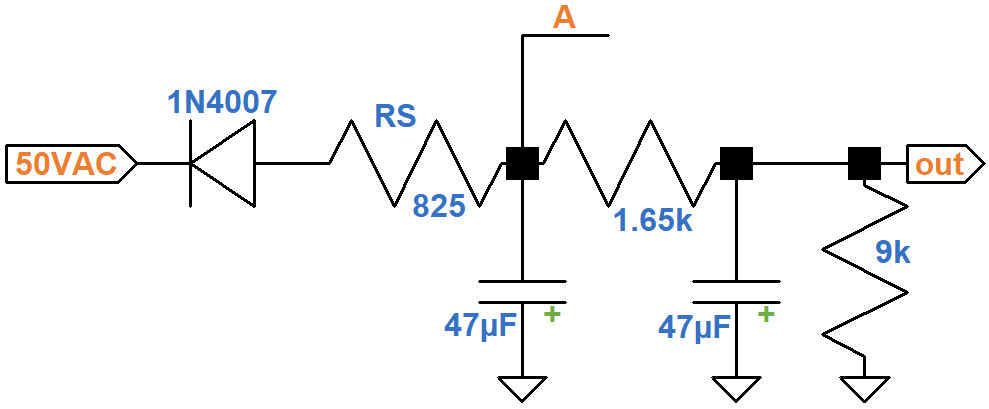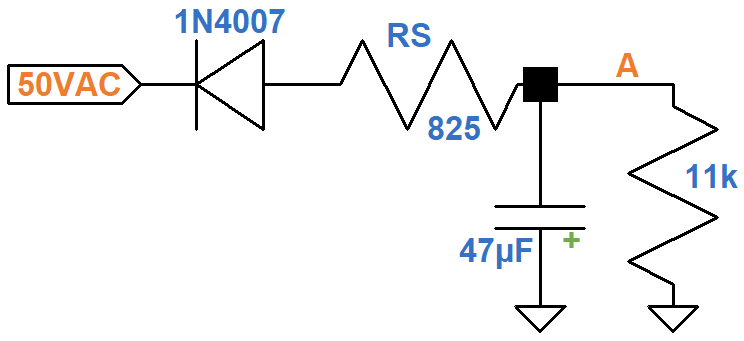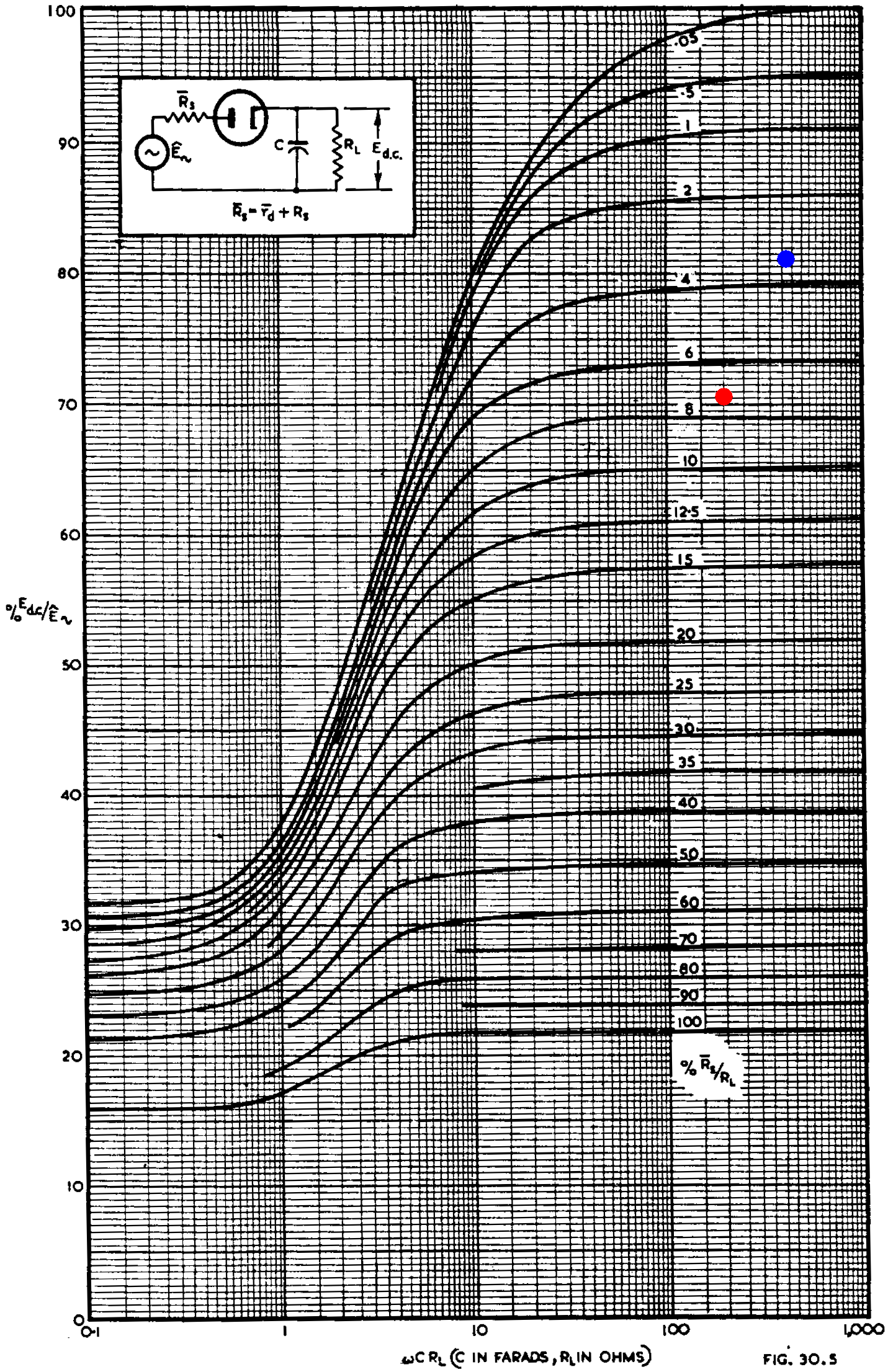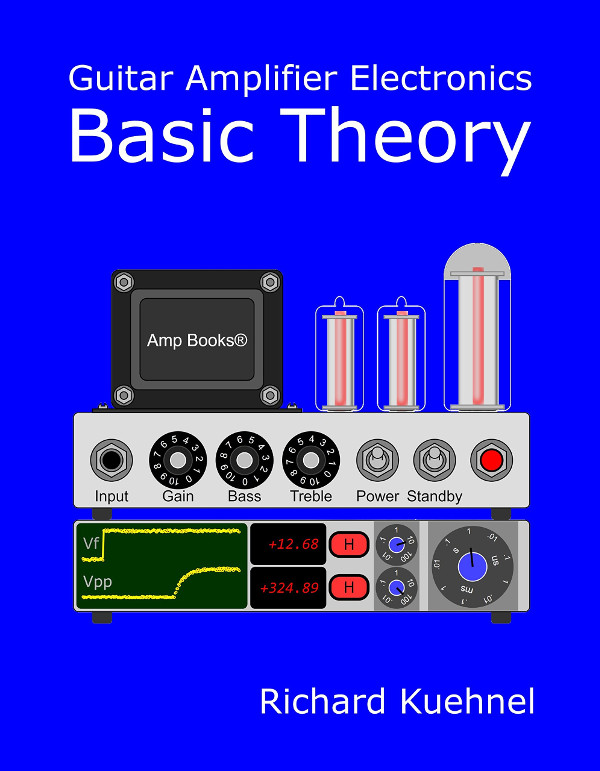The Mesa/Boogie Dual Rectifier has a grid bias supply that is switchable to support EL34 power pentodes or 6L6 beam power tetrodes. Here is EL34 mode.For 6L6 mode, resistor R1 is switched out to create a shunt resistance of 22kΩ. When switched in, the effective shunt resistance of the parallel resistors is 9kΩ.

The power transformer 50VAC secondary tap drives the grid supply via a 1N4007 diode and series resistor RS to provide negative voltage pulses for subsequent ripple filtering. (Notice that the electrolytic capacitors are oriented so that their positive terminals are grounded, which is not their usual orientation.) Let's consider EL34 mode. This creates the least negative grid bias, with only 9kΩ between the output and ground. The 3.3kΩ resistors in parallel create an effective resistance of 1.65kΩ.The power tube grids draw very little current, so the load resistance between node A and ground is

RL = 1.65kΩ + 9kΩ = 11kΩ

If we ignore the second capacitor, the circuit looks like the one for a half-wave rectifier with a capacitor input filter described by the Radiotron Designer's Handbook.1The circuit is shown in the upper left corner of these curves.For 60Hz commercial power, a 47μF capacitor, and an 11kΩ load, the value needed for the X axis is

2π(60Hz)(47μF)(11kΩ) = 195

Radiotron's graph includes transformer winding resistance in the value of RS, which we can ignore. With RS = 825Ω, the ratio of RS to RL is

825Ω / 11kΩ = 0.075

An X axis value of 195 intercepts an imagined curve for 7.5 percent at the red dot. The Y axis indicates that the ratio of DC output voltage to AC peak input voltage is 71 percent. Transformer secondary voltages are in RMS,2 so to get the peak AC input voltage we multiply by the square root of 2:

(50V)(1.414) = 70.7V

There is about 0.7V of forward voltage across the diode, creating 70V peak in front of RS. The magnitude of the DC voltage at node A is therefore

(70V)(0.71) = 49.7V

The output voltage is attenuated by the voltage divider formed by the 1.65kΩ and 9kΩ resistances:

(49.7V)(9kΩ) / (9kΩ + 1.65kΩ) = 42V

The DC grid bias is therefore -42V. Mesa/Boogie measures -39V. Repeating these steps with R1 switched out gives us the blue dot and a DC grid bias of -49V. Mesa/Boogie measures -51V.

#### References

F. Langford-Smith, ed., Radiotron Designer's Handbook, 4th ed., (Harrison: RCA, 1953), p. 1172.

2Richard Kuehnel, Guitar Amplifier Electronics: Basic Theory, (Seattle: Amp Books, 2018), pp. 154-156.New! A deep dive into the electronic design of the ultimate high-gain rig-of-death!From system design concepts to individual stage operation, an all-new examination of Bassman electronics.From "woody" to narrow panel to brownface to blackface Reverb, an in-depth exploration of Fender Deluxe circuitry.Master the basics of preamp, power amp, and power supply design.Design your amp using a structured, professional methodology.Know it works by measuring performance at every point in the amplifier.